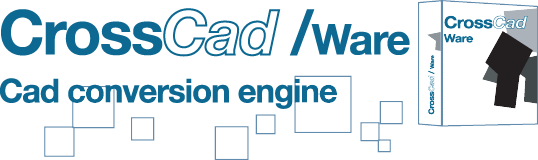CrossCad/Ware allows software vendors to integrate Datakit technology into their own application.

Datakit libraries provide you a full-documented Application Programming Interface (API) to enable interoperability among CAD systems.

## Why use Datakit libraries ?

Libraries are an efficient solution for software companies that currently integrate Datakit solutions for CAD data exchange as components to their own software.
These libraries allow access to the information stored in native data files such as 3D & 2D geometrical and topological data and all the concepts of dimensions, tolerances, features, and annotations

## Datakit proposes 2 different ways to cooperate with software companies :

Both methods are transparent to the end user.

###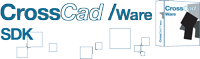Fully integrated transfers into the vendors software solution: conversion of all entities one by one including data attributes processing. This gives a powerful grasp on the transferred data. This solution meets the needs of most of Datakit customers (Software companies) that seek to go beyond mere file-to-file transfer with sophisticated capability for processing data attributes.

• 3DVIA / CATIA / SOLIDWORKS Composer
• 3Shape DCM
• ACIS
• CATIA V4 3D
• CATIA V5 2D
• CATIA V5 3D
• CATIA V6 / 3DEXPERIENCE 2D
• CATIA V6 / 3DEXPERIENCE 3D
• Cercon
• CEREC - Sirona Dental
• CGM 2D
• CGR
• Creo View
• DWG / DXF 2D
• DWG / DXF 3D
• FBX
• glTF
• IFC
• IGES
• Inventor
• JT
• LAVA 3M
• OBJ
• Parasolid
• PLMXML
• Procera
• ProE / Creo Parametric 2D
• ProE / Creo Parametric 3D
• Revit
• Rhino
• SMG 3D
• Solid Edge 2D
• Solid Edge 3D
• SOLIDWORKS 2D
• SOLIDWORKS 3D
• STEP
• STL
• UG NX 2D
• UG NX 3D
• VDA

• 3DXML
• 3MF
• ACIS
• CATIA V5 3D
• CGR
• FBX
• glTF
• IFC
• IGES
• JT
• OBJ
• Parasolid
• PDF 3D
• PRC
• SOLIDWORKS 3D
• STEP
• STL
• UG NX 3D

###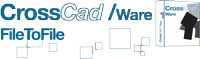File-to-file transfers using intermediary files  an easy solution to test the robustness and the capabilities of the components. Then, if software companies need functionalities that are specific to their software, they realise the full advantage of using CrossCad/Ware SDK libraries.

## Inputs

• 3DVIA / CATIA / SOLIDWORKS Composer
• 3Shape DCM
• ACIS
• CATIA V4 2D
• CATIA V4 3D
• CATIA V5 2D
• CATIA V5 3D
• CATIA V6 / 3DEXPERIENCE 2D
• CATIA V6 / 3DEXPERIENCE 3D
• CEREC - Sirona Dental
• CGR
• Creo View
• DWG / DXF 2D
• DWG / DXF 3D
• FBX
• glTF
• IFC
• IGES
• Inventor
• JT
• OBJ
• Parasolid
• PLMXML
• Procera
• ProE / Creo Parametric 2D
• ProE / Creo Parametric 3D
• Revit
• Rhino
• Solid Edge 2D
• Solid Edge 3D
• SOLIDWORKS 2D
• SOLIDWORKS 3D
• STEP
• STL
• UG NX 2D
• UG NX 3D
• VDA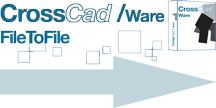## Outputs

• 3DXML
• 3MF
• ACIS
• CATIA V4 3D
• CATIA V5 3D
• CGR
• DXF
• EMF
• FBX
• glTF
• IFC
• IGES
• JT
• OBJ
• Parasolid
• PDF 2D
• PDF 3D
• PRC
• SOLIDWORKS 3D
• STEP
• STL
• UG NX 3D
• Unisurf
• VDA
• VRML
 Outputs Inputs 3DXML 3MF ACIS CADDS CATIA V4 3D CATIA V5 3D CGR COLLADA FBX glTF IFC IGES JT OBJ Open CASCADE Parasolid PDF 3D PRC Robcad SOLIDWORKS 3D STEP STL UG NX 3D Unisurf X X X X X X X X X X X X X X X X X X X X X X X X X X X X X X X X X X X X X X X X X X X X X X X X X X X X X X X X X X X X X X X X X X X X X X X X X X X X X X X X X X X X X X X X X X X X X X X X X X X X X X X X X X X X X X X X X X X X X X X X X X X X X X X X X X X X X X X X X X X X X X X X X X X X X X X X X X X X X X X X X X X X X X X X X X X X X X X X X X X X X X X X X X X X X X X X X X X X X X X X X X X X X X X X X X X X X X X X X X X X X X X X X X X X X X X X X X X X X X X X X X X X X X X X X X X X X X X X X X X X X X X X X X X X X X X X X X X X X X X X X X X X X X X X X X X X X X X X X X X X X X X X X X X X X X X X X X X X X X X X X X X X X X X X X X X X X X X X X X X X X X X X X X X X X X X X X X X X X X X X X X X X X X X X X X X X X X X X X X X X X X X X X X X X X X X X X X X X X X X X X X X X X X X X X X X X X X X X X X X X X X X X X X X X X X X X X X X X X X X X X X X X X X X X X X X X X X X X X X X X X X X X X X X X X X X X X X X X X X X X X X X X X X X X X X X X X X X X X X X X X X X X X X X X X X X X X X X X X X X X X X X X X X X X X X X X X X X X X X X X X X X X X X X X X X X X X X X X X X X X X X X X X X X X X X X X X X X X X X X X X X X X X X X X X X X X X X X X X X X X X X X X X X X X X X X X
 Outputs Inputs DXF X X X X X X X X X X X X X X X X X

## CrossCad/Ware : a recognized, complete and robust product

Datakit collaborates as an OEM with more than 100 vendors. The company is now starting to operate on a market where customers are upgrading existing solutions.

Historically Datakit moved from offering standalone application targeting end-users to components embedded inside CAD systems. This tendency, started in 2000, was clearly confirmed these past years. Supplying components accounts for 70% of Datakit revenues.

New customers work in the fields of design, reverse engineering, creation of interactive documentation, viewing, rendering and QC.

Data exchange impacts an increasing range of disciplines. This makes it natural and imperative to work with vendors to enable as many end-users as possible to exchange their CAD data.

Satisfaction requires deep and close relationship between supplier and customers. Datakit has the ability to listen and propose solution and will make sure its solutions will meet your needs now as well as tomorrow.

Its success in making a software module compared to developing an independent application relies on its ability to focus on breaking down the entities into the finest grained detail possible and its building structures that enable data to be stored thoroughly and then used as needed by different applications.

Its independence, the skills it has on board, and its ability to listen to customers and propose solutions definitively explain the company’s success. On the technical level it is able to support metadata, in addition to purely geometric information. Actually, it is increasingly dealing with issues such as the management of assemblies, annotations and tolerancing data. Basically all the data that comes under the headings FD&T and PDM.

## Related documents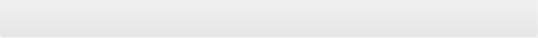Client room